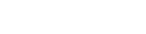# Javascript reduce()

## Syntax

``````
array.reduce(callbackFunction, initialValue);

``````

There are two parameters to the reduce() method -

• A callback function callbackFunction. The function is also known as a reducer.
• Initial value, which is optional.

Lets take an example of reduce function. Here we are adding elements of an array. For this, we usually use a for loop to iterate over the items and add them up, as seen in the following example.

## Example

``````
let sum = 0;

const numbers = [10, 20, 30];

for(let i = 0; i < numbers.length; i++) {
sum += numbers[i];
}

console.log(sum);

``````
##### Output
``````
60

``````

Now we will use the reduce function to do the same. Reduce function makes it possible to reduce an array to a single value.

## Example

``````
const sum = 0;

const numbers = [10, 20, 30];

const reducer = (total, item) => {
};

const value = numbers.reduce(reducer, sum)
console.log(value)

``````
##### Output
``````
60

``````